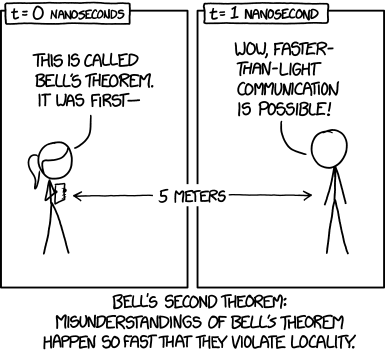### Quantum computing perspective for (Big) Data management

Valter Uotila

January 30, 2023

Second year PhD student at the Unified Database Management Systems research group

Supervised by professors Jiaheng Lu and Jukka K. Nurminen

My previous focus was in category theory applied to multi-model databases -- now the topic is extended with quantum computing

### Quantum computing and information processingPlethora of different kinds of computing devices and computing models besides ordinary CPUs

and quantum computers.

#### Quantum computing works on various hardware

Although the hardware varies the abstract model of quantum computing is quite standard (circuit):

#### Why does it work?

The abstract foundation of quantum computing is based on quantum mechanics.

See section Introduction to quantum mechanics in book Quantum Computation and Quantum Information 10th Anniversary Edition by Nielsen and Chuang.

#### Approaching from machine learning perspective

You have developed a machine learning model to classify cats and dogs.

Model outputs that the image has 80% prob of represeting cat and 20% prob of representing a dog.

#### Approaching from machine learning perspective

If you modify the model, you want:

the prob of cat + the prob of dog = 100%

#### Making things more abstract:

Instead of cats and dogs, we have vectors.

Instead of probabilites, we have complex numbers which implicitly encode the probabilities.

##### Single qubit systems

$|\psi\rangle = \alpha|0\rangle + \beta|1\rangle$

where $\alpha,\beta \in \Complex$ are amplitudes and

$|\alpha|^2 + |\beta|^2 = 1$.

The states $|0\rangle = \begin{bmatrix} 1 \\ 0 \end{bmatrix}$ and $|1\rangle = \begin{bmatrix} 0 \\ 1 \end{bmatrix}$ are called computational basis.

##### Multiple qubits

\begin{aligned} |\psi\rangle &= (a_0|0\rangle + b_0|1\rangle) \otimes (a_1|0\rangle + b_1|1\rangle) \\ & = a_0a_1|00\rangle + a_0b_1|01\rangle + b_0a_1|10\rangle + b_0b_1|11\rangle. \end{aligned}

We see that $n$ qubit system has $2^n$ amplitudes (i.e. the coefficients).

##### Evolution of quantum systems

Initial system of qubits (usually $|00\ldots0\rangle$)

Apply gates the qubits. Gates are modelled as unitary matrixes. The idea is that the sum of the squared norms of complex amplitudes is preserved.

Measure the system. The system ends up to some classical state. The sequence of classical bits is the result of the quantum computation.

### Superposition

One qubit can be in multiple states simultaneously.

For example, the most common method to put a qubit to a superposition is to apply Hadamar-gate

$H|0\rangle = \frac{1}{\sqrt{2}}\begin{bmatrix} 1 & 1 \\ 1 & -1 \end{bmatrix}\begin{bmatrix} 1 \\ 0 \end{bmatrix} = \frac{1}{\sqrt{2}} \begin{bmatrix} 1 \\ 1 \end{bmatrix} = \frac{|0\rangle + |1\rangle}{\sqrt{2}}$.

### Entanglement

Entanglement roughly means that two or more qubits are connected and they can interact in ways such that the state of each qubit cannot be described independently of the state of the other qubits.

For example, the most common method to entangle two qubits is to use CNOT gate:

CNOT$(|x\rangle \otimes |y\rangle) = |x\rangle \otimes |x + y \mod 2\rangle$.

### Some challenges in quantum information processing

#### that are related to "data"### No-cloning theorem

Theoretically provable theorem that quantum states cannot be copied or cloned.

See Box 12.1: The no-cloning theorem in book Quantum Computation and Quantum Information.

### Measuring destroys states

Measuring a qubit collapses it to either 0 or 1. After the measurement we cannot modify the measured qubit anymore.

### Error

Quantum computing is never performed in absolutely isoleted system and the environment creates decoherence and other quantum noise.

We can tackle the error by developing quantum error correction.

## Implementation byInitial paper in Nature: Quantum annealing with manufactured spins

## Motivation for quantum annealing

D-wave has many examples of use cases:

## General idea

Goal: finding the global minimum of a given objective function

## Simulated annealing on classical hardware## Annealing process: bias## Annealing process: couplers## Relationship to circuit-model

### What kind of database problems are we tackling with quantum computing?

The research plan published in: Uotila, Valter . Synergy between Quantum Computers and Databases . in Proceedings of the VLDB 2022 PhD Workshop co-located with the 48th International Conference on Very Large Databases (VLDB 2022), 05/09/2022 . http://ceur-ws.org/Vol-3186/paper_1.pdf

Solving classical database optimization and modeling problems with quantum computing

Problem: quantum computing might not scale and bring any benefits

### SQL2Circuits: Predicting metrics for SQL queries with quantum natural language processing method

Transformation of SQL queries into parametrized circuits based on their context-free grammar representation

Optimizing circuit parameters so that the framework could predict execution times and cardinalities

### Optimizing task and virtual machine allocation

Quantum annealing -based QUBO formulation for task and virtual machine allocation to physical machines in data centers

We are taking into account energy consumption and sustainability metrics

Uotila, Valter and Lu, Jiaheng. Quantum Annealing Method for Dynamic Virtual Machine and Task Allocation in Cloud Infrastructures from Sustainability Perspective. SMDB 2023 Workshop. In review.

### Join order optimization

One of the most classical database problems: find the optimal way to join relational tables

Already has a QUBO formulation but the formulation is not spesific for this problem: we aim to find specific QUBO formulation

#### As a conclusion, quantum computing will not yet reshape database field

The current hardware has limited capabilites.

But quantum computing offers many interesting promises which we research:

• computational speedup
• new methods to develop and design novel algorithms
• hybrid frameworks
• quantum machine learning is a big topic
• probably lower energy consumption

### Thank you for your interest and the opportunity to give a presentation!